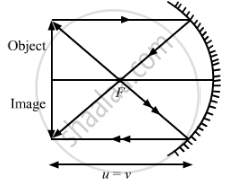Share

# A Spherical Mirror Produces an Image of Magnification -1 on a Screen Placed at a Distance of 50 Cm from the Mirror. - CBSE Class 10 - Science

ConceptLens Formula and Magnification

#### Question

A spherical mirror produces an image of magnification -1 on a screen placed at a distance of 50 cm from the mirror.

(a) Write the type of mirror.

(b) Find the distance of the image from the object.

(c) What is the focal length of the mirror?

(d) Draw the ray diagram to show the image formation in this case.

#### Solution

(a) As magnification is negative, the image formed is real.

Hence, it is a concave mirror.

(b) m=-v/u=-1

therefore u=v=-50" cm"

"Distance of the image from the object ="|u|+|v|=100" cm"

(c) By using mirror formula:-

1/f=1/v+1/u=1/((-50))+1/((-50))=-1/25

therefore f=-25" cm"

(d)Is there an error in this question or solution?

#### Video TutorialsVIEW ALL 

Solution A Spherical Mirror Produces an Image of Magnification -1 on a Screen Placed at a Distance of 50 Cm from the Mirror. Concept: Lens Formula and Magnification.
S